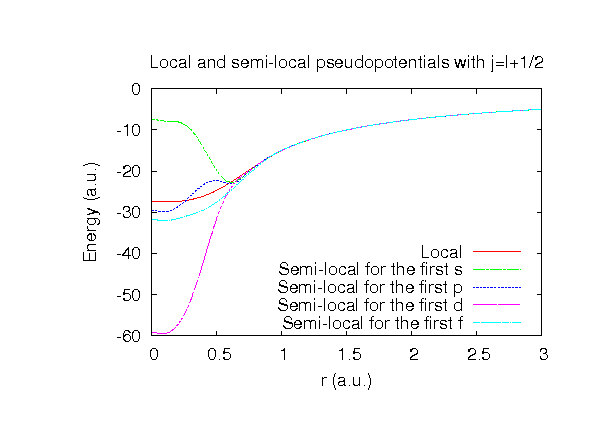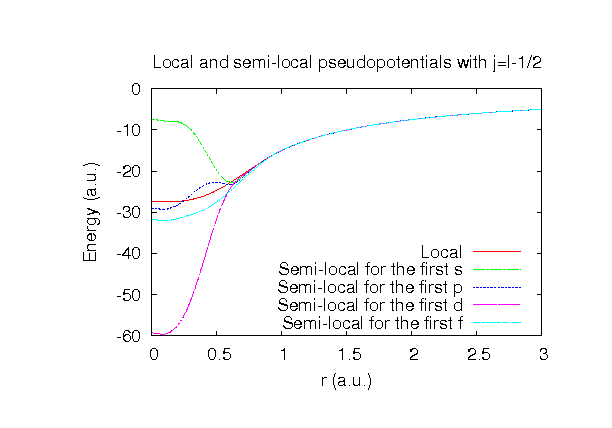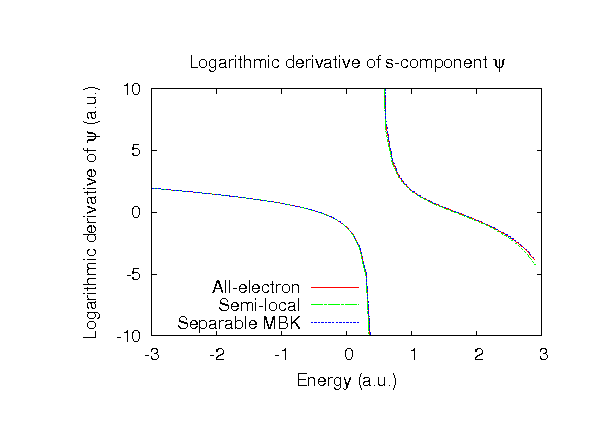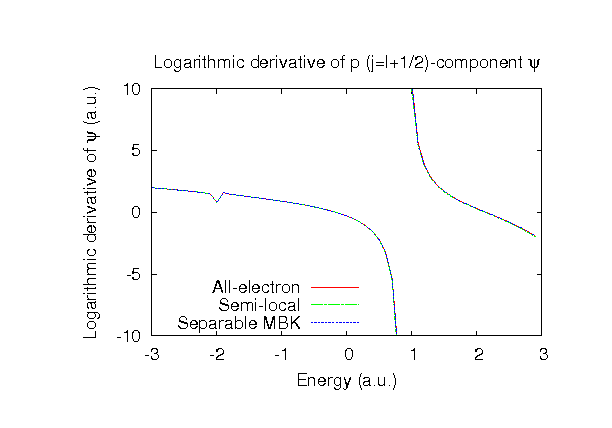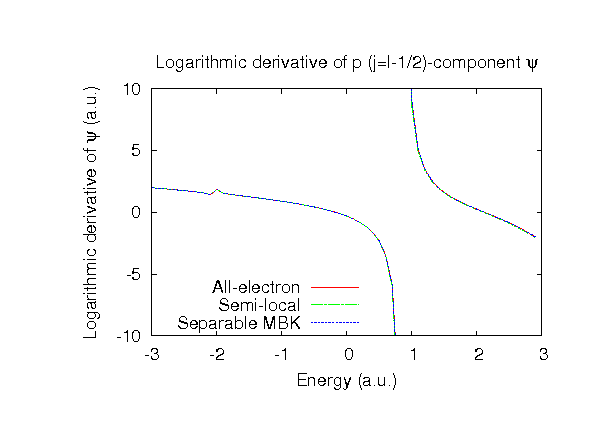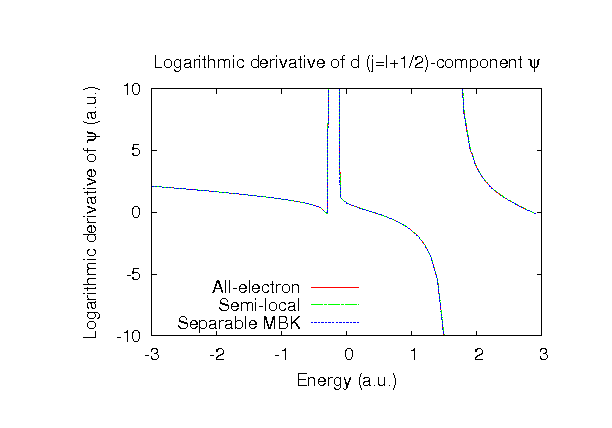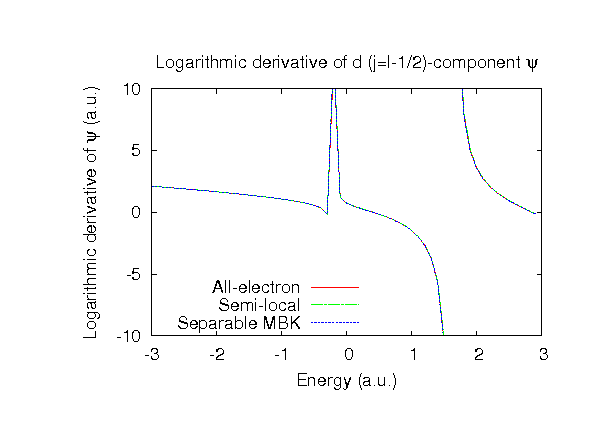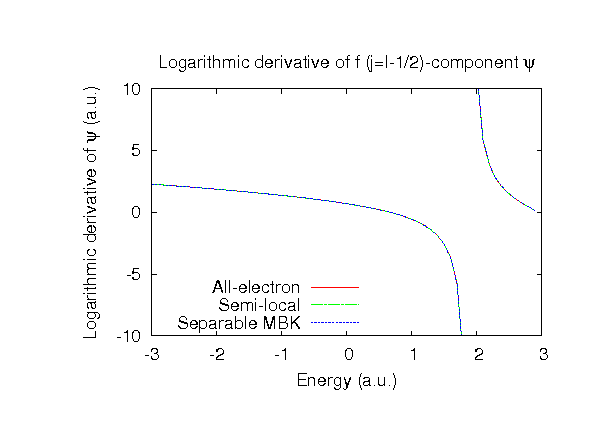### Fully relativistic pseudopotentials

Fully relativistic pseudopotentials generated by the MBK (PRB 47, 6728 (1993)) scheme within LDA (CA13) and GGA (PBE13) which contain a partial core correction and fully relativistic effects including spin-orbit coupling.

### Pseudo-atomic orbitals

The number below the symbol means a cutoff radius (a.u.) of the confinement potential. These file includes fifteen radial parts for each angular momentum quantum number l (=0,1,2,3). The basis functions were generated by variationally optimizing the corresponding primitive basis functions in the single atom, the dimer, and a MnO molecule. The input files used for the orbital optimization can be found at Mn_opt.dat , Mn2_opt.dat , and MnO_opt.dat . Since Mn_CA13.vps and Mn_PBE13.vps include the 3s, 3p, 3d, and 4s states (15 electrons) as the valence states, the minimal basis set is Mn*.*-s2p1d1. Our recommendation for the choice of cutoff radius of basis functions is that Mn6.0.pao is enough for bulks, but Mn8.0.pao or Mn10.0.pao is preferable for molecular systems.

### Benchmark calculations by the PBE13 pseudopotential with the various basis functions

(1) Calculation of the total energy as a function of lattice constant in the fcc structure, where the total energy is plotted relative to the minimum energy for each case. a0 and B0 are the equilibrium lattice constant and bulk modulus obtained by fitting to the Murnaghan equation of state. The difference between Mn6.0-s3p3d3 and Mn6.0-s3p3d3f1 in the total energy at the minimum point is 0.020 eV/atom. An input file used for the OpenMX calculations can be found at Mnfcc-EvsV.dat . For comparison the result by the Wien2k code is also shown, where the calculation was performed by default setting in the Ver. 10.1 of Wien2k except for the use of RMT x KMAX of 14.(2) Calculations of the band dispersion in the fcc structure, where the non-spin polarized collinear calculation with the lattice constant of 3.50 Ang. was performed using Mn_PBE13.vps and Mn6.0-s3p3d3, and the origin of the energy is taken to be the Fermi level. The input file used for the OpenMX calculations can be found at Mnfcc-Band.dat . For comparison the result by the Wien2k code is also shown, where the calculation was performed by default setting in the Ver. 10.1 of Wien2k except for the use of RMT x KMAX of 14.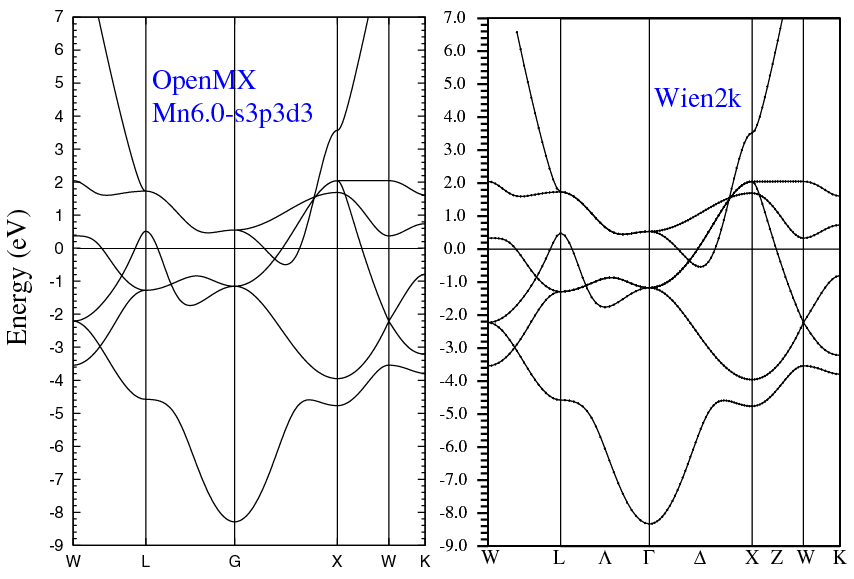(3) Calculations of a MnO molecule, where Mn_PBE13.vps, O_PBE13.vps, Mn10.0-s3p3d3, Mn10.0-s3p3d3f1, and O7.0-s3p3d2 were used. The input files used for the OpenMX calculations can be found at MnO_O1.dat , MnO_Mn1.dat , MnO_O2.dat , MnO_Mn2.dat , MnO_O_cp1.dat , MnO_Mn_cp1.dat , MnO_O_cp2.dat , and MnO_Mn_cp2.dat ,

 Equilibrium bond length (Ang.) Dipole moment (Debye) Atomization energy (eV) Atomization energy (couterpoise corrected) (eV) Mn10.0-s3p3d3, O7.0-s3p3d2 1.634 4.68 4.99 4.98 Mn10.0-s3p3d3f1, O7.0-s3p3d2 1.634 4.68 5.01 5.00 Other calc. 1.635 a 4.99a Expt. 1.648b 3.70b

a W.A. Al-Saidi, H. Krakauer, and S. Zhang, Phys. Rev. B 73, 075103 (2006).
b H.P. Loock, B. Simard, S. Wallin, and C. Linton, J. Chem. Phys. 109, 8980 (1998).

### Supplementary information for the GGA (PBE13) pseudopotential